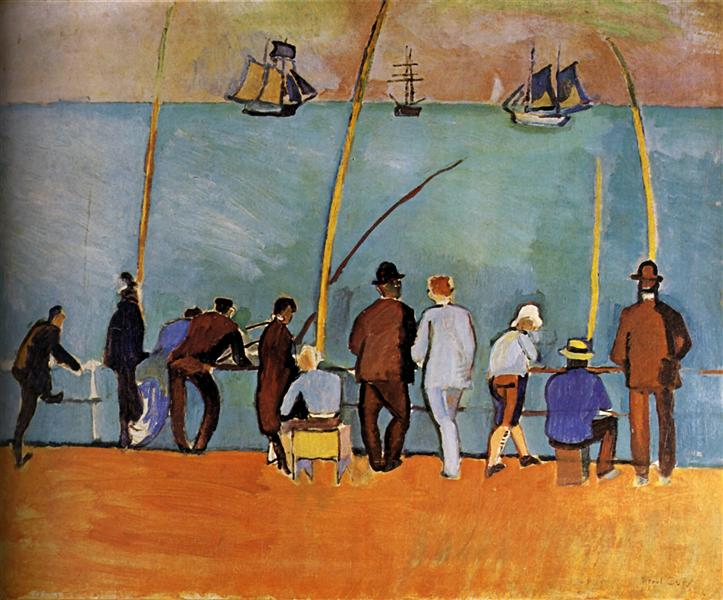# The Poisson distribution and log-linear models

First, a video.

This week you will:

• learn about log-linear models
• learn about over-dispersion
• when there is more error than you expect

# A Model for Counts: FishingAnglers by Raoul Dufy

Imagine sitting on the banks of the river Seine in Paris, fishing. Fish swim past randomly at a constant rate. If we catch fish for an hour, how many fish do we catch? Because they swim past randomly, the number will vary. it will also depend on the density of fish (and how fast they swim etc. etc.). But if we catch them at rate $$\mu$$, the mean number we catch in time $$t$$ will be $$\lambda = \mu t$$. The actual number will vary, and will follow a Poisson distribution:

$Pr(N=r | \lambda) = \frac{\lambda^r e^{-\lambda}}{r!}$

Now, as some of you may know, the French for fish is poisson (and the French call April 1st poisson d’avril, so beware). But the Poisson distribution is actually named after Baron Siméon Denis Poisson.